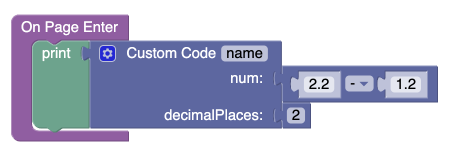# Codeless Substraction is wrong

Hi,

I am using a service in Codeless to do some calculation.I am trying to do a simple substraction and the result is wrong. For instance, 2.2 - 1.2 gives me 1.0000000000000002. ANy idea ?

Thanks.

Hi,

Codeless is based on JavaScript. JavaScript treats decimals as floating point numbers, which means operations like addition might be subject to rounding error.

Regards,
Olha

1 Like

Thanks for your answer. Do you have any solution to solve my problem ?

Here’s an option:The `Custom Code` block has the following logic:

The code as text is:

``````    var p = Math.pow(10, decimalPlaces);
return Math.round(num * p) / p;
``````

Other alternative approaches can be found here:

Regards,
Mark